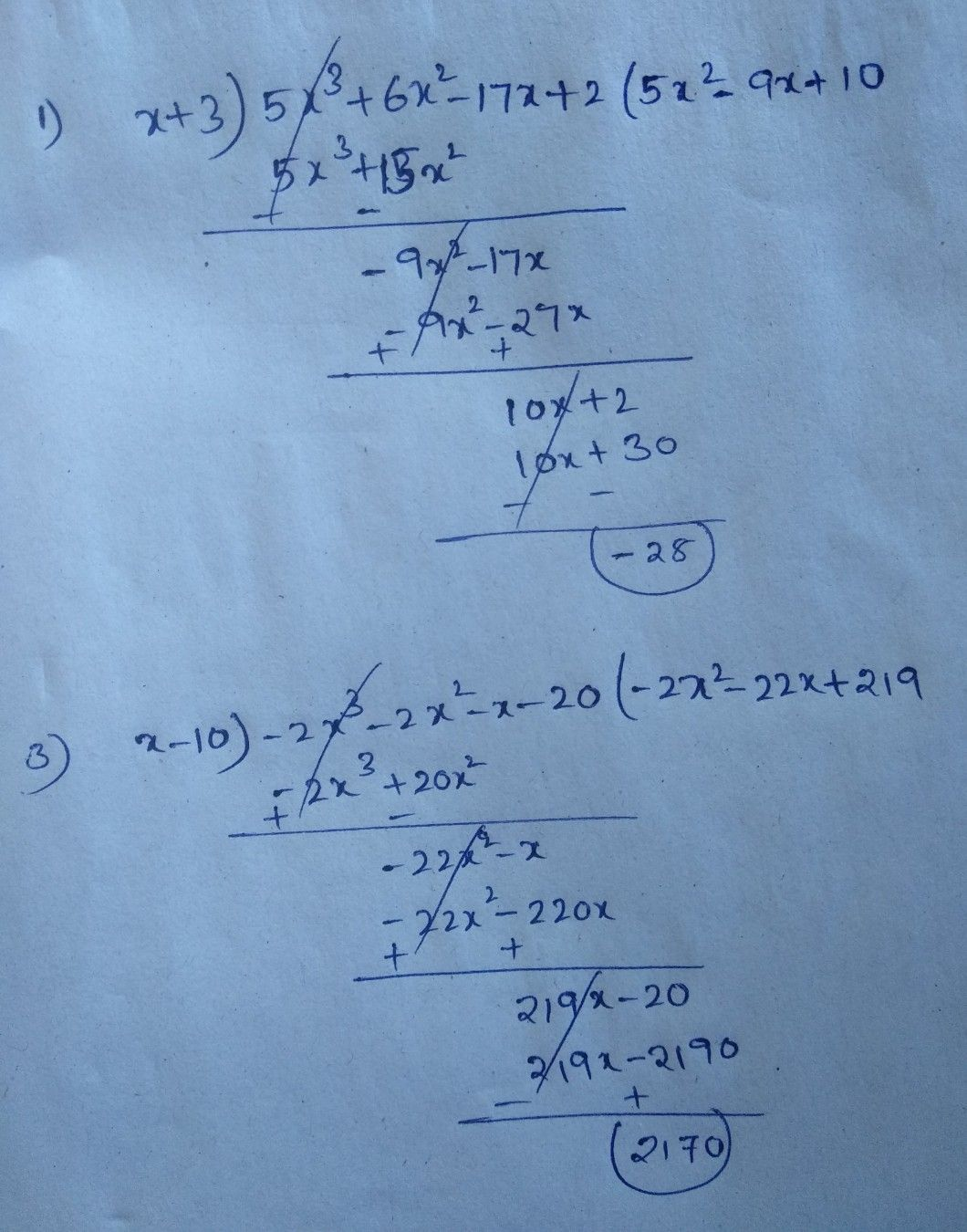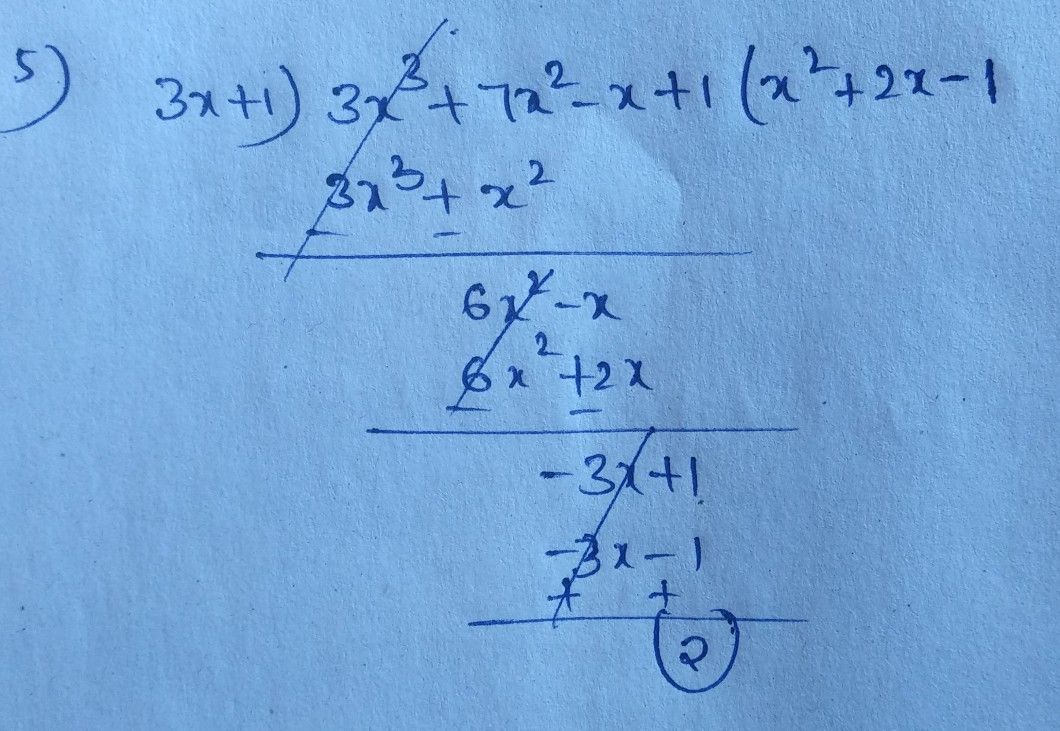Symbol
ProblemDivide using $long$ division or synthetic d. $1.$ $\left(5x^{3}+6x^{2}-17x+2\right)\div \left(x+3\right)$ $3$ $\left(-2x^{3}-2x^{2}-x-20\right)\div \left(x-10\right)$ $5.$ $\left(3x^{3}-x+1+7x^{2}\right)\div \left(3x+1\right)$
10th-13th grade
Other
Search count: 105
SolutionQanda teacher - saaketh